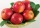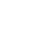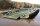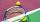# Greatest common divisor (GCD) - math word problems

#### Number of problems found: 55

• Largest squaresHow many of the largest square sheets did the plumber cut the honeycomb from 16 dm and 96 dm?
• Identical cubesFrom the smallest number of identical cubes whose edge length is expressed by a natural number, can we build a block with dimensions 12dm x 16dm x 20dm?
• Children's homeThe children's home received a gift to Nicholas of 54 oranges, 81 chocolate figurines, and 135 apples. Every child received the same gift and nothing was left. a) How many packages could be prepared? b) what did the children find in the package?
• Profitable companyThree businessman decide to open up their own company. They agree to distribute the yearly profits made in the same ratio as their initial investments. They invest R 50 000, R 75 000 and R25 000, respectively. The profit made by the company in the first y
• Length of a stringWhat is the smallest length of a string that we can cut into 18 equal parts and even 27 equal parts (in decimeters)?
• A largeA large gear will be used to turn a smaller gear. The large gear will make 75 revolutions per minute. The smaller gear must make 384 revolutions per minute. Find the smallest number of teeth each gear could have. [Hint: Use either GCF or LCM. ]
• Three linesAt 6 am, three bus lines are departing from the station. The first line has an interval of 24 minutes. The second line has an interval of 15 minutes. The third line runs at regular intervals of more than 1 minute. The third line runs at the same time as t
• QuotientFind quotient before the bracket - the largest divisor 51 a + 34 b + 68 121y-99z-33
• Common divisorsFind all common divisors of numbers 30 and 45.
• Cutting paperDivide a rectangular paper with dimensions 220mm and 308mm into squares of the same size so that they are as large as possible. Specify the length of the side of the square.
• Long bridgeRoman walked on the bridge. When he heard the whistle, he turned and saw running Kamil at the beginning of the bridge. If he went to him, they would meet in the middle of the bridge. Roman, however, rushed and so did not want to waste time returning 150m.
• Wide fieldThe field is 203 meters wide 319 meter long, what is the greater length of the rope by which length and width can be measured and find the exact number of time.
• Glass panelA rectangular glass panel with dimensions of 72 cm and 96 cm will cut the glazier on the largest square possible. What is the length of the side of each square? How many squares does the glazier cut?
• Reminder and quotientThere are given the number C = 281, D = 201. Find the highest natural number S so that the C:S, D:S are with the remainder of 1,The children of the tennis school received 64 white and 48 yellow balls from the sponsor. When asked about how many balls they could take, they were answered: "You have so many that none of you will have more than 10 balls and all will have the same numbe
• Seven numbersWrite seven 4-digit numbers that are divisible by 3 and at the same time by 4.
• LCD 2The least common denominator of 2/5, 1/2, and 3/4
• Four poplarsFour poplars are growing along the way. The distances between them are 35 m, 14 m, and 91 m. At least how many poplars need to be dropped to create the same spacing between the trees? How many meters will it be?
• GardenThe garden has the shape of a rectangle measuring 19m20cm and 21m60cm. Mr. Novák will fence it. It wants the distance between adjacent pillars to be at least two meters and a maximum of three meters. He would also like the distances between the adjacent p
• Ornamental shrubsChildren committed to plant 240 ornamental shrubs. Their commitment however exceeded by 48 shrubs. Write ratio of actually planted shrubs and commitment by lowest possible integers a/b.

Do you have an interesting mathematical word problem that you can't solve it? Submit a math problem, and we can try to solve it.

We will send a solution to your e-mail address. Solved examples are also published here. Please enter the e-mail correctly and check whether you don't have a full mailbox.

Please do not submit problems from current active competitions such as Mathematical Olympiad, correspondence seminars etc...

Do you want to calculate greatest common divisor two or more numbers?